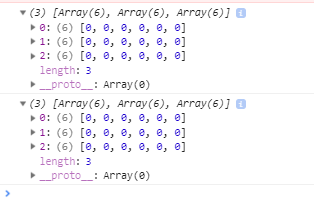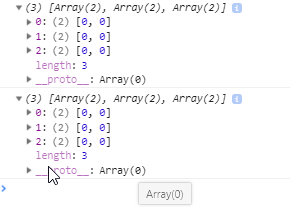# Re initializing Variables Inside a Loop

Tell us what’s happening:

I can’t fix this problem. I don’t really understand what kind of changes should be in matrix variable.

``````
function zeroArray(m, n) {
// Creates a 2-D array with m rows and n columns of zeroes
let newArray = [];
let row = ;
for (let i = 0; i < m; i++) {
// Adds the m-th row into newArray

for (let j = 0; j < n; j++) {
// Pushes n zeroes into the current row to create the columns
row.push(0);
}

// Pushes the current row, which now has n zeroes in it, to the array
newArray.push(row);
}
return newArray;
}

let matrix = zeroArray(3,2);
console.log(matrix);
``````

User Agent is: `Mozilla/5.0 (Windows NT 6.1; Win64; x64) AppleWebKit/537.36 (KHTML, like Gecko) Chrome/68.0.3440.106 Safari/537.36`.

If you open the developer console (Ctrl-Shift-I in Chrome) and run the tests, you’ll seein the console. Notice that the each of the three arrays created have six elements instead of two. What variable would you need to re-initialize (i.e., make empty) to have only two elements for each array each time, as shown below?Removed screenshot of ES6 solution.

This is my first post. Forgive me if I confused matters with my screenshot.

So after clicking on the link provided…might I suggest breaking the problem into 2 parts.
First, make a single row array produce the required number of 0 elements.
Then, embed the solution to the first step into another iterative loop to replicate the single row solution across multiple rows of the array.